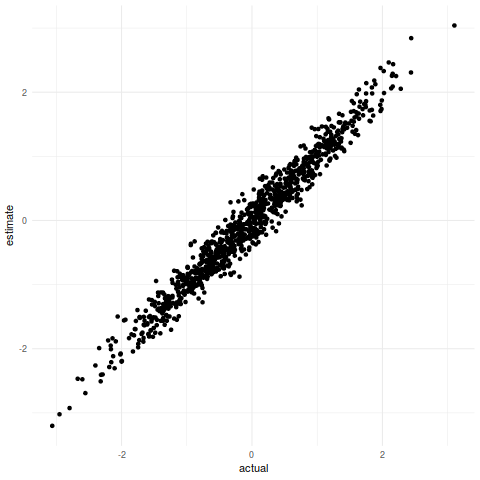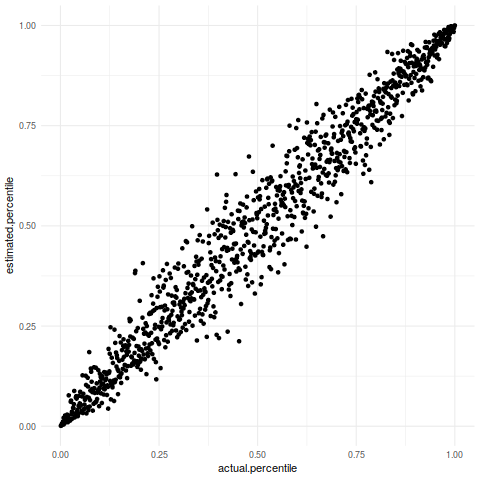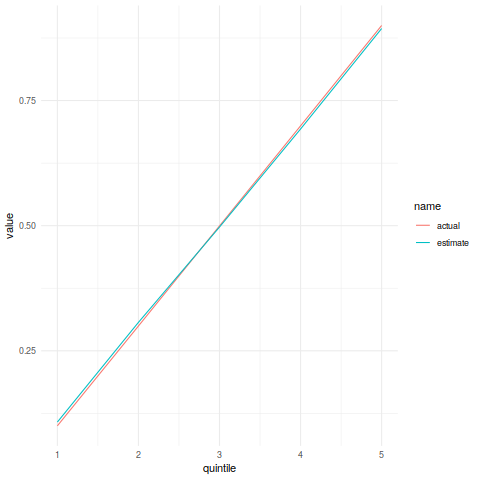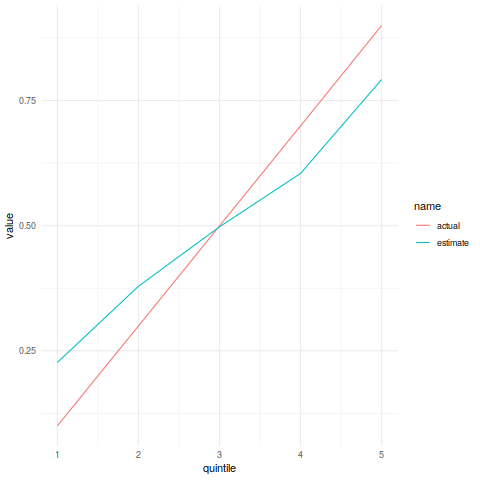# Is Dunning-Kruger just an inevitable outcome of their experiment setup?

Posted on July 17, 2020

I’ve been reading the Wikipedia page for Dunning-Kruger effect. There are some surprising things in it that I’ve detailed in this tweet thread. I think most people are mitaken about what Dunning and Kruger’s work actually tells us.

But that isn’t what I’m writing about here. Wikipedia also has a section that suggests, maybe, the experimental findings are mathematically inevitable given how the experiment was setup.

The intuition here is that if you are in, for example, the 96th percentile and you are asked to estimate your rank then you can only overestimate by 4 percent but you can underestimate by a lot more than that. The same idea is also true for those in the 4th percentile except there they have a lot more space to overestimate rather than underestimate.

I’m going to make a simulation to investigate this.

``````## Start by generating a sample of people's *actual* competance
scores <- data.frame(actual=rnorm(1000))

## Then simulate them estimating their competence
## The estimates are centred on their true competence with a bit of variation
## either way
## NB: There is no link between the precision of the estimate and competence
scores\$estimate <- rnorm(1000, mean=scores\$actual, sd=0.2)

library(ggplot2)

ggplot(scores,aes(x=actual,y=estimate)) +
geom_point() + theme_minimal()
``````The weirdness happens when we convert this to a percentile view:

``````perc.rank <- function(x) trunc(rank(x))/length(x)

scores\$actual.percentile <- perc.rank(scores\$actual)
scores\$estimated.percentile <- perc.rank(scores\$estimate)

ggplot(scores,aes(x=actual.percentile,y=estimated.percentile)) +
geom_point() + theme_minimal()``````In the first plot the “compression” was mostly in the middle, which makes sense because that is where most of the people are but in the percentile plot there are areas of high density in the corners.

Dunning and Kruger grouped their people into percentiles based on competence and then compared the results

``````library(dplyr)
library(tidyr)

## I really hope there is a better way to calculate quintiles!
scores %>% mutate(quintile=ifelse(actual.percentile<0.2,1,
ifelse(actual.percentile<0.4,2,
ifelse(actual.percentile<0.6,3,
ifelse(actual.percentile<0.8,4,5)
)
)
)) -> scores

scores %>% group_by(quintile) %>% summarise(actual=mean(actual.percentile),
estimate=mean(estimated.percentile)
)``````
quintile actual estimate
1 0.1 0.107492462311558
2 0.2995 0.306765
3 0.4995 0.49724
4 0.6995 0.69315
5 0.9 0.89392039800995
``````scores %>% group_by(quintile) %>%
summarise(actual=mean(actual.percentile),
estimate=mean(estimated.percentile)) %>%
pivot_longer(cols=c("actual","estimate")) %>%
ggplot(aes(x=quintile,y=value,color=name)) + geom_line() + theme_minimal()``````This is a similar result to Dunning-Kruger, but the effect is much smaller.

I can probably make a bigger effect by increasing the standard deviation of the competence estimates.

``````scores\$estimate <- rnorm(1000, mean=scores\$actual, sd=1)
scores\$estimated.percentile <- perc.rank(scores\$estimate)
scores %>% group_by(quintile) %>% summarise(actual=mean(actual.percentile),
estimate=mean(estimated.percentile)
)``````
quintile actual estimate
1 0.1 0.22664824120603
2 0.2995 0.378895
3 0.4995 0.497655
4 0.6995 0.60444
5 0.9 0.792034825870647
``````scores %>% group_by(quintile) %>%
summarise(actual=mean(actual.percentile),
estimate=mean(estimated.percentile)) %>%
pivot_longer(cols=c("actual","estimate")) %>%
ggplot(aes(x=quintile,y=value,color=name)) + geom_line() + theme_minimal()``````This is a bit more similar to the actual paper but the result is still a long way from the original.

despite test scores that placed them in the 12th percentile, the participants estimated they ranked in the 62nd percentile

In the simulation, the bottom quintile participants do not rank themselves as more competent than the median participant.

This shows that a result in the same direction as Dunning-Kruger but no where near as strong comes just from how the experiment is setup.